# What Is a Tape Diagram?

## What Is a Tape Diagram?

A tape diagram is a rectangular drawing that appears like a tape piece with divisions to support mathematical calculations. It is a graphic tool used commonly in solving ratio-based mathematical word problems.

Tape diagrams are visual representations that represent the sections of a ratio by using rectangles. As they are a visual model it takes attention to detail to draw them. They break down complex mathematical word problems and help simplify it. They are depicted in the form of a strip or as a piece of tape. Tape diagrams are also commonly called a bar model, a length model, a strip diagram, or a fraction strip.

## Uses of Tape Diagrams

A tape diagram at its core is a simplistic approach to a complicated mathematical problem. It is commonly used by teachers to help teach their students better. The diagram aids teachers by allowing them to introduce the element of fun to learning. Meanwhile, tape diagrams make it easier for students to struggle with mathematical problems to find out what calculations are necessary to solve word problems. What's more, tape diagrams can also be used to provide better and efficient learning environments where students can learn different approaches to complex problems. In particular, they are easy for the modeling of mathematical operations like addition, subtraction, multiplication, division, fractions, and proportions.

## Benefits of Using a Tape Diagram

The advantage of the tape diagram is the students won't memorize concepts they don't understand. The tape diagram is used to effectively solve a mathematical word problem involving -

• Model ratios when the units are similar in both quantities
• Discuss more complex comparison problems
• Three items ratio
• Two different ratios are compared
• Complex word problems

## How To Use Tape Diagrams To Solve Problem?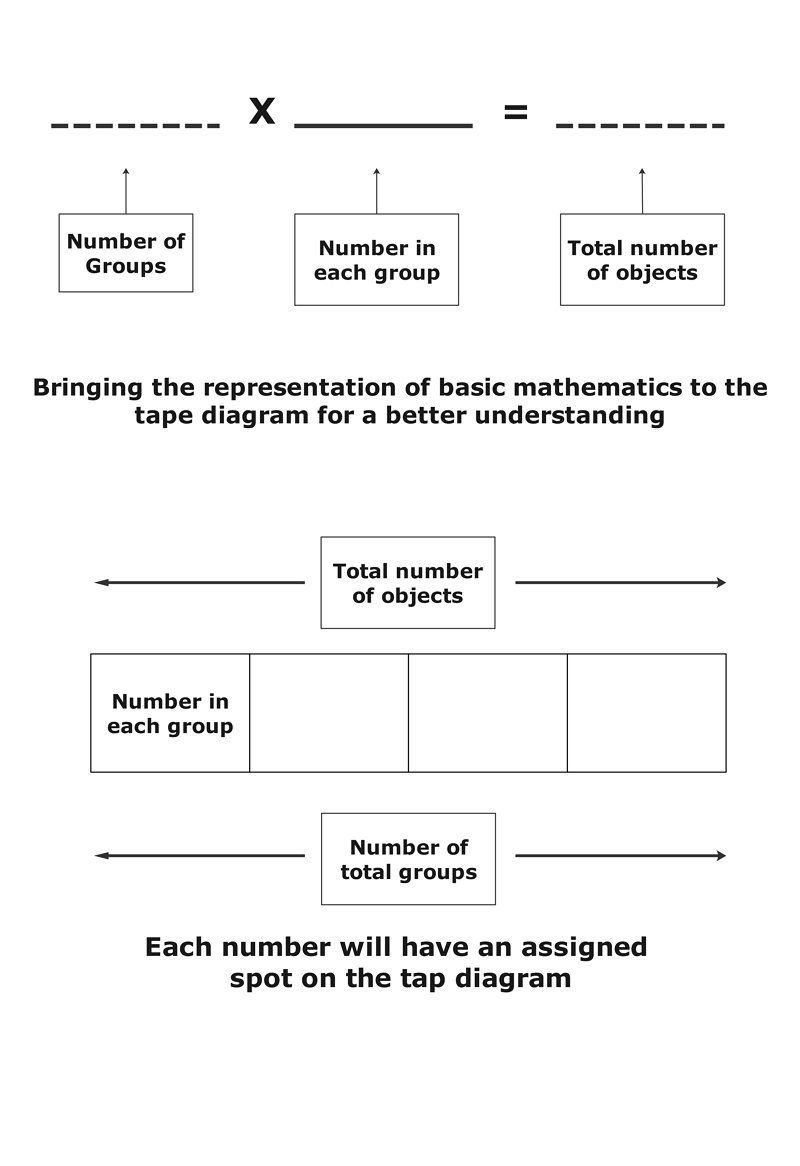Using this visual model students can easily solve mathematical problems as it provides a bird's eye perspective to comparative mathematical equations. When presented with a problem to students you can follow the simple steps listed below -

• Based on the given problem, a horizontal rectangle is drawn and cut into sections
• These sections represent groups of numbers in the mathematical problem
• This helps students get a visual look at the problem and helps them easily solve it
• Then students can perform the mathematical operation as defined in the problem

## Example of Tape DiagramThe above example, ratio comparison of how much money individuals have made is represented. Tom has two boxes Jimmy has three and whereas Susan has 5 boxes. The total is \$1200. That is denoted by brackets encompassing the tape diagram. Each box/rectangle is \$120.

The tape diagram provides an easy way to calculate the mathematical problem. It is visible the amount of money each individual has made. Tape diagrams are ideal for these calculations. This way the ratio can be calculated, effectively.

## How To Draw a Tape Diagram Using EdrawMax?

• Step 1 Open EdrawMax -> and select a blank canvas.
•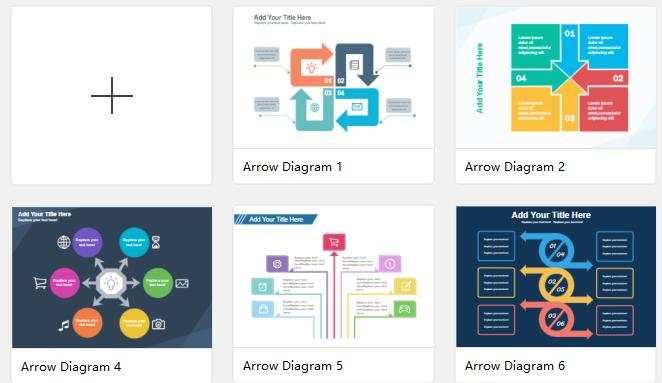• Step 2 Use the wide range of symbols from the libraries available to create your tape diagram.
•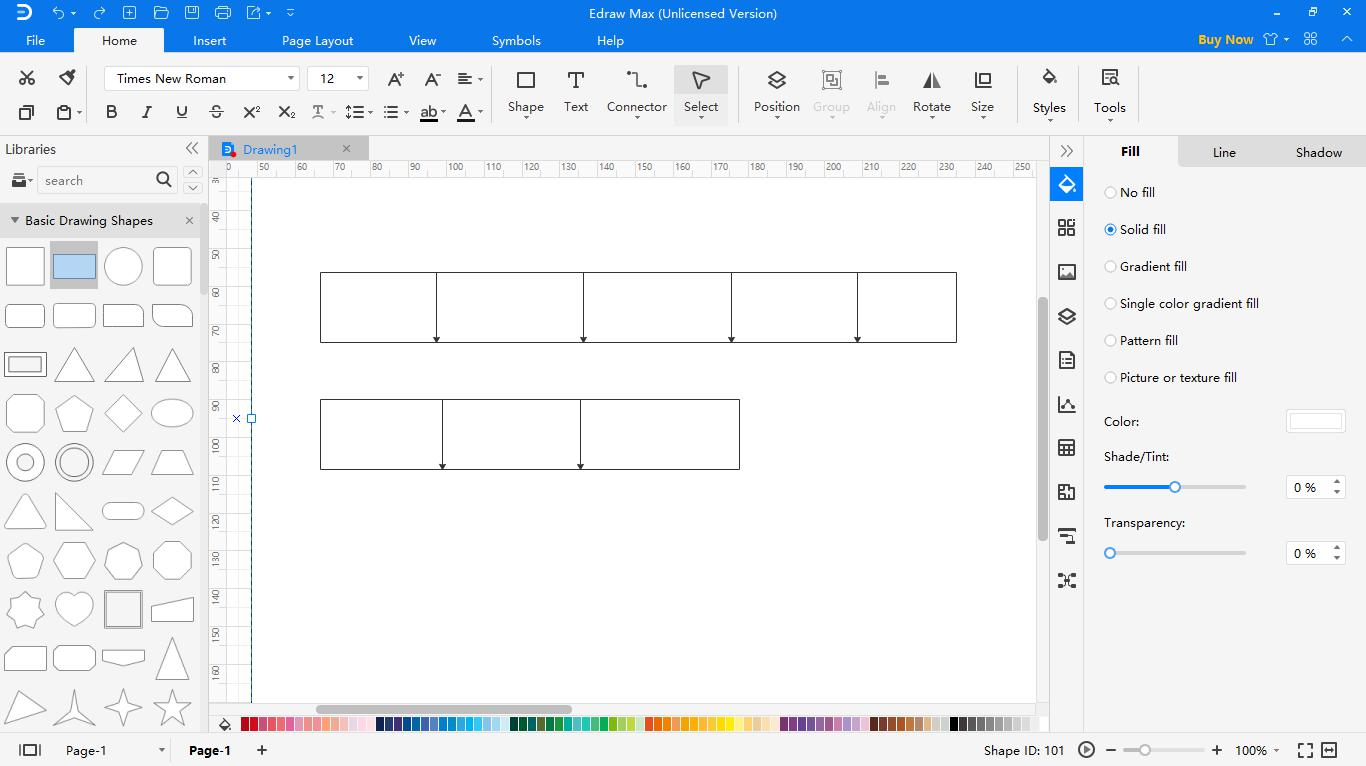• Step 3 Add in your text and design the tape diagram to suit the requirements. You can also icons from the multitude of options available.
•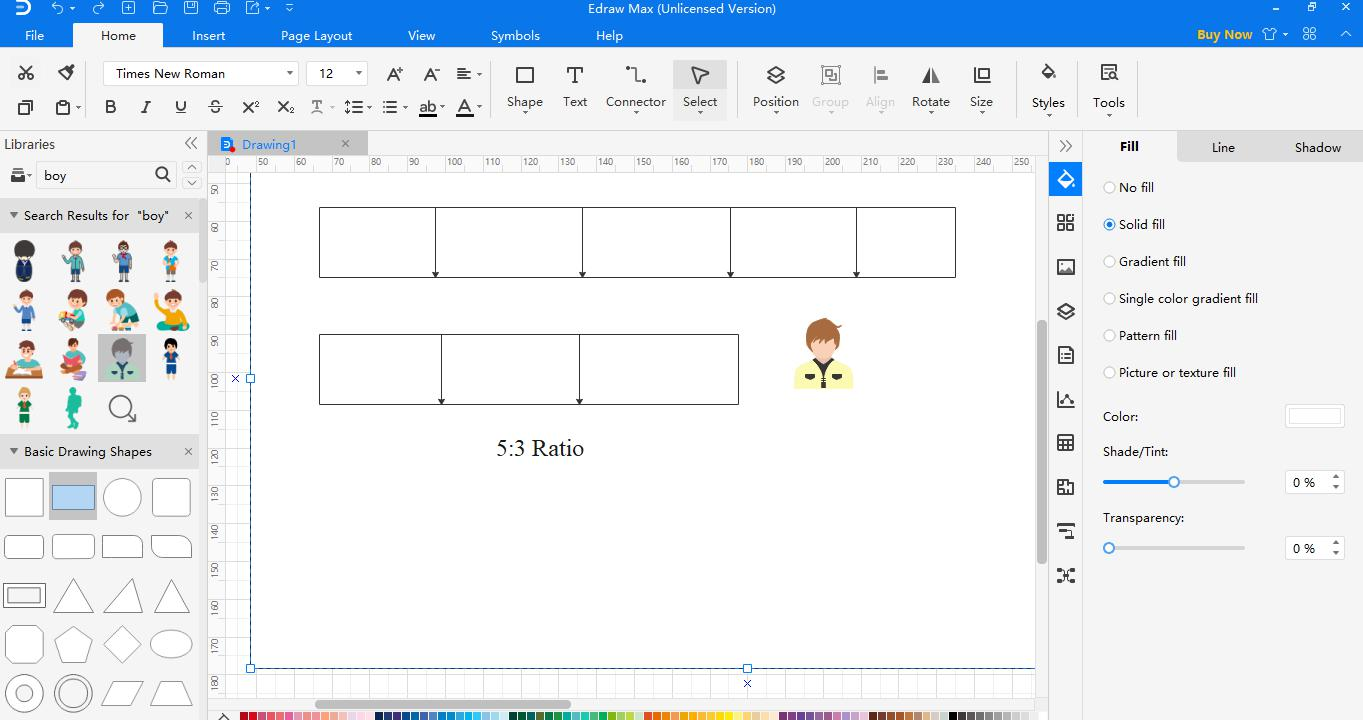• Step 4 Save and export your diagram to any file type you wish to. EdrawMax is compatible with multiple file types, such as Microsoft Office, Graphs, PS, PDF, HTML, SVG, Visio.
•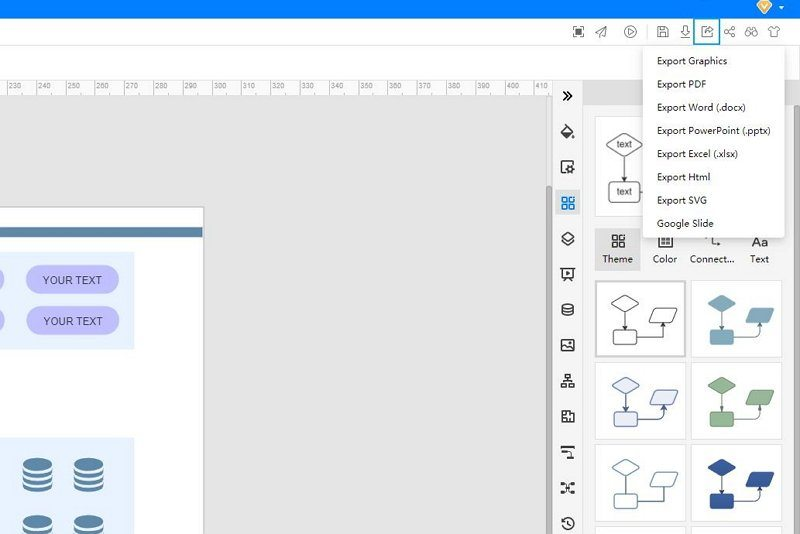## Conclusion

The tape diagram is a format to quickly solve mathematical calculations. The advantage of the tape diagram is that the students won't memorize concepts they don't understand. If they know how to do it or how to adapt it to various situations, a memorized guideline can't benefit a pupil. On the other hand, the tape diagrams are basic visual aids that allow students to understand a problem better. They will become more effective problem-solvers by using them.

EdrawMax online is a conceptual diagramming program that helps construct the flowcharts, hierarchical charts, mind maps, network diagrams, floor plans, and tape diagrams. All shapes and clipart are vector format design, which means your tape diagram will never get blurred, irrespective of what size you change it to. EdrawMax online is a diagram creator and is a full-featured application commonly adopted for making these diagrams. It is extremely user-friendly and offers 100% privacy when it comes to security.

### Related Articles